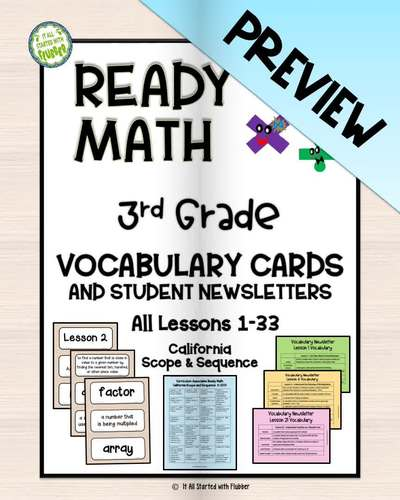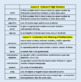# 3rd Grade Ready Math Vocabulary Cards - CA Scope and SequenceSubject
Resource Type
Product Rating
4.0
(1 Rating)
File Type

PDF

(233 MB|168 pages)
Product Description

Our bestselling Ready Math Vocabulary Cards for 3rd Grade now have a sister product for the 2019 California scope and sequence! We've added a vocabulary newsletter for parents and students for every lesson. Please check the list below to make sure this resource matches your program.

You'll love using these vocabulary cards with students - use as a station, to introduce the lesson, as a review of the lesson, for students needing more support, for your ELL students, and for test prep. All cards are labeled with the lesson number for easy sorting and storage and are ready to print.

In addition to the vocabulary cards, we've included a master list of all lessons specific to the updated CA program, a teacher list of all vocabulary terms and definitions, and a handy half-page newsletter to send home with students at the start of each lesson.

Here is the scope and sequence of the 2019 CA program used to create this product:

Lesson 1 - Use Place Value to Round Numbers

Lesson 2 - Add 3-Digit Numbers

Lesson 3 - Subtract 3-Digit Numbers

Lesson 4 - Understand the Meaning of Multiplication

Lesson 5 - Multiply with 0, 1, 2, 5, and 10

Lesson 6 - Multiply with 3, 4, and 6

Lesson 7 - Multiply with 7, 8, and 9

Lesson 8 - Use Order and Grouping to Multiply

Lesson 9 - Using Place Value to Multiply

Lesson 10 - Understand the Meaning of Division

Lesson 11 - Understand How Multiplication and Division Are Connected

Lesson 12 - Multiplication and Division Facts

Lesson 13 - Understand Patterns

Lesson 14 - Understand Area

Lesson 15 - Multiply to Find Areas

Lesson 17 - Solve 1-Step Word Problems Using Multiplication and Division

Lesson 18 - Solve 2-Step Word Problems Using All Four Operations

Lesson 19 - Scaled Graphs

Lesson 20 - Understand What a Fraction Is

Lesson 21 - Understand Fractions on a Number Line

Lesson 22 - Understanding Equivalent Fractions

Lesson 23 - Finding Equivalent Fractions

Lesson 24 – Understand Comparing Fractions

Lesson 25 – Use Symbols to Compare Fractions

Lesson 26 – Measure Length and Plot Data on Line Plots

Lesson 27 - Time

Lesson 28 – Liquid Volume

Lesson 29 - Mass

Lesson 30 – Understand Categories of Shapes

Lesson 32 – Area and Perimeter of Shapes

Lesson 33 – Partition Shapes into Parts with Equal Areas

Please look for our CA vocabulary assessments - coming soon!!

Remember to leave us feedback on your purchase to earn TpT credits to use for future purchases. Also, we'd love for you to click to "follow me" — and be sure to look for us on social media!

Thank you for your interest in our resources.

Total Pages
168 pages
N/A
Teaching Duration
N/A
Report this Resource to TpT
Reported resources will be reviewed by our team. Report this resource to let us know if this resource violates TpT’s content guidelines.